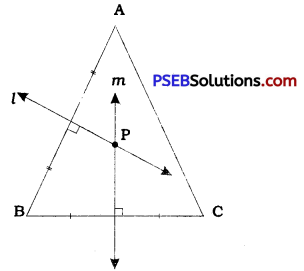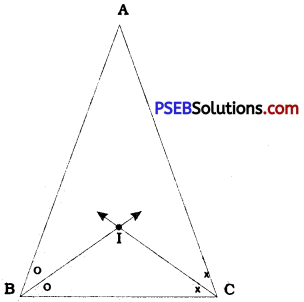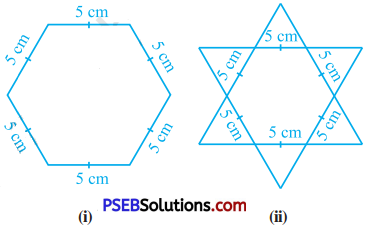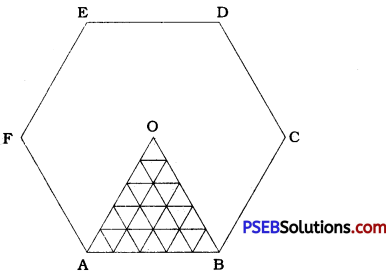# PSEB 9th Class Maths Solutions Chapter 7 Triangles Ex 7.5

Punjab State Board PSEB 9th Class Maths Book Solutions Chapter 7 Triangles Ex 7.5 Textbook Exercise Questions and Answers.

## PSEB Solutions for Class 9 Maths Chapter 7 Triangles Ex 7.5

Question 1.
ABC is a triangle. Locate a point in the interior of ∆ ABC which is equidistant from all the vertices of ∆ ABC.In ∆ ABC, draw l, the perpendicular bisector of side AB and m, the perpendicular bisector of side BC. Name the point of intersection of l and m as P.
P is a point on the perpendicular bisector of AB. Hence, P is equidistant from A and B.
∴ PA = PB
P is a point on the perpendicular bisector of BC. Hence, P is equidistant from B and C.
∴ PB = PC
Thus, PA = PB = PC
Hence, P is the required point which is equidistant from all the vertices of ∆ ABC.
Note: Since ∆ ABC given here is an acute angled triangle, point P lies in the interior of ∆ ABC. If ∆ ABC is a right angled Mangle, point P lies on the hypotenuse. Actually, in that case, point P will be the midpoint of the hypotenuse. Lastly, if ∆ ABC is an obtuse angled triangle, point P lies in the exterior of ∆ ABC. This point P is called the circumcentre of ∆ ABC.Question 2.
In a triangle locate a point in its interior which is equidistant from all the sides of the triangle.In ∆ ABC, draw the bisectors of ∠B and ∠C to intersect each other at point I.
I is a point on the bisector of ∠ B. Hence, I is equidistant from sides BA and BC. Similarly, I is a point on the bisector of ZC. Hence, I is equidistant from sides BC and CA.
Thus, point I is the required point which is equidistant from all the three sides AB, BC and CA of ∆ ABC.
This point I is called the incentre of ∆ ABC. It always lies in the interior of ∆ ABC irrespective of the type of ∆ ABC.

Question 3.
In a huge park, people are concentrated at three points (see the given figure):A: where there are different slides and swings for children.
B: near which a man-made lake is situated.
C: which is near to a large parking and exit.
Where should an ice cream parlour be set up so that maximum number of persons can approach it ?
(Hint: The parlour should be equidistant from A, B and C.)
First of all, construct ∆ ABC with the given points A, B and C as vertices. Then, as shown in example 1, draw the perpendicular s bisectors of any two sides of ∆ ABC and name their point of intersection as P.

Now, the ice cream parlour should be set up at the location given by point P as it is equidistant from all the three places (points) A, B and C.Question 4.
Complete the hexagonal and star shaped < Rangolies [see figure (1) and (2)] by filling them with as many equilateral triangles of > side 1 cm as you can. Count the number < of triangles in each case. Which has more triangles?